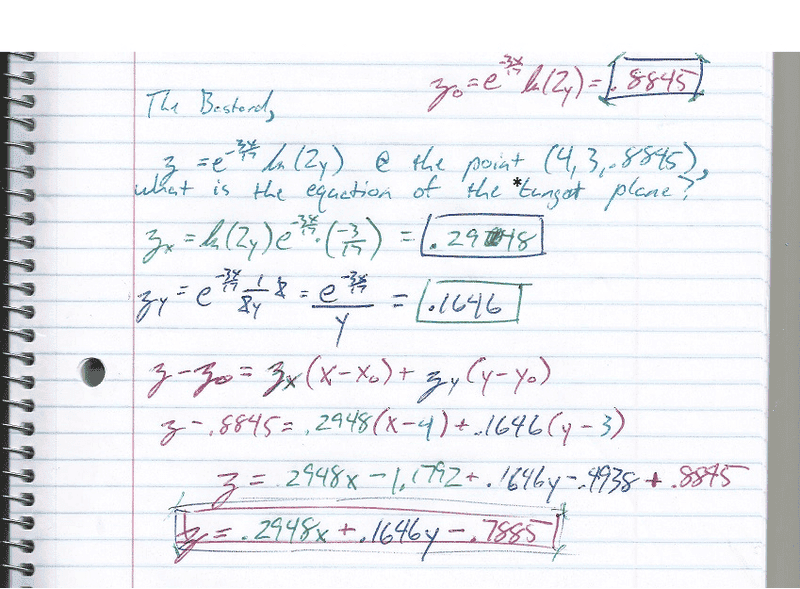# What is the equation of a tangent plane for the following values

Gold Member

## The Attempt at a SolutionSo I am not sure how many times I tried this thing but the final answer is still wrong. I am sure it is something simple, where did I goof it?

*and why do we call it a tangent plane?

## Answers and Replies

dirk_mec1
I can't really read it can you write it out in tex?

Gold Member
I can't really read it can you write it out in tex?

Just bought some new color pens so that can make my notes a bit 'loud' although they are fun to write! :)

The problem is probably just my arithmetic so really isn't that big of a deal.

What I would like to go over is the 'tangent plane'. It seems the formula is just an extension from point slope form in ℝ2 brought onto 3 dimensional surfaces by taking advantage of the derivatives along each axis (x and y for this case). Let's take a look at it,

(z-zo) = zx(x-xo)+zy(y-yo)

We know we can calculate this equation to get 'z' but it would seem we could also do more with it if we have information for 'z', it's leading coefficient, and the point 'z0' to work out information on the other side of the equation. Very cool.

It is wonderful that this translates so well from point slope form and it would seem working with more dimensions (even if we can't 'see' them) would be pretty straightforward so long as when setting our equation = to 'z' (or whatever) our leading coefficient is divided through. Good stuff!